PSAT Math : How to find the area of a circle

Example Questions

← Previous 1 3 4

Example Question #6 : How To Find The Area Of A Circle

If a circle has circumference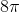, what is its area?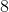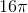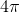Explanation:

If the circumference is, then since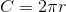we know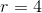. We further know that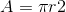, so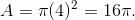Example Question #7 : How To Find The Area Of A Circle

If the equation of a circle is (x – 7)+ (y + 1)= 81, what is the area of the circle?

6561π

81π

18π

49π

81π

Explanation:

The equation is already in a circle equation, and the right side of the equation stands for r2 → r= 81 and r = 9

The area of a circle is πr2, so the area of this circle is 81π.

Example Question #8 : How To Find The Area Of A Circle

Assume π = 3.14

A man would like to put a circular whirlpool in his backyard. He would like the whirlpool to be six feet wide. His backyard is 8 feet long by 7 feet wide. By state regulation, in order to put a whirlpool in a backyard space, the space must be 1.5 times bigger than the pool. Can the man legally install the whirlpool?

No, because the area of the backyard is smaller than the area of the whirlpool.

Yes, because the area of the whirlpool is 28.26 square feet and 1.5 times its area would be less than the area of the backyard.

No, because the area of the backyard is 30 square feet and therefore the whirlpool is too big to meet the legal requirement.

No, because the area of the whirlpool is 42.39 square feet and 1.5 times its area would be greater than the area of the backyard.

Yes, because the area of the whirlpool is 18.84 square feet and 1.5 times its area would be less than the area of the backyard.

Yes, because the area of the whirlpool is 28.26 square feet and 1.5 times its area would be less than the area of the backyard.

Explanation:

If you answered that the whirlpool’s area is 18.84 feet and therefore fits, you are incorrect because 18.84 is the circumference of the whirlpool, not the area.

If you answered that the area of the whirlpool is 56.52 feet, you multiplied the area of the whirlpool by 1.5 and assumed that that was the correct area, not the legal limit.

If you answered that the area of the backyard was smaller than the area of the whirlpool, you did not calculate area correctly.

And if you thought the area of the backyard was 30 feet, you found the perimeter of the backyard, not the area.

The correct answer is that the area of the whirlpool is 28.26 feet and, when multiplied by 1.5 = 42.39, which is smaller than the area of the backyard, which is 56 square feet.

Example Question #9 : How To Find The Area Of A Circle

There are two identical circles on a plane that overlap. The radius of both circles is 1. The region in which they overlap has an area of π.

What is the total area of the 2 overlapping circles?

2π

0

π

2

1

π

Explanation:

The total area of both circles is π + π = 2π

Since the region overlaps, we cannot count it twice, so we must subtract it.

we get 2π – π = π

Example Question #10 : How To Find The Area Of A Circle

A square with a side length of 4 inches is inscribed in a circle, as shown below. What is the area of the unshaded region inside of the circle, in square inches?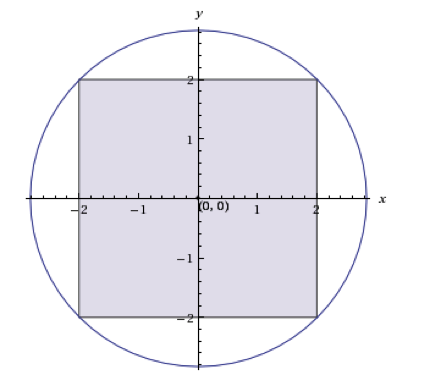4π-4

8π-4

8π - 16

8π-8

2π-4

8π - 16

Explanation:

Using the Pythagorean Theorem, the diameter of the circle (also the diagonal of the square) can be found to be 4√2.  Thus, the radius of the circle is half of the diameter, or 2√2.  The area of the circle is then π(2√2)2, which equals 8π.  Next, the area of the square must be subtracted from the entire circle, yielding an area of 8π-16 square inches.

Example Question #1 : How To Find The Area Of A Circle

If a circle has a circumference of 16π, what would its area be if its radius were halved?

16π

64π

16π

Explanation:

The circumference of a circle = πd where d = diameter.  Therefore, this circle’s diameter must equal 16.  Knowing that diameter = 2 times the radius, we can determine that the radius of this circle = 8.  Halving the radius would give us a new radius of 4.  To find the area of this new circle, use the formula A=πr² where r = radius.  Plug in 4 for r.  Area will equal 16π.

Example Question #2 : How To Find The Area Of A Circle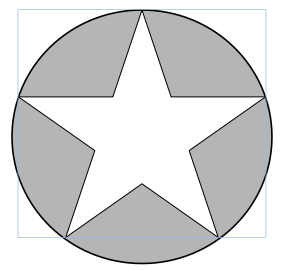A star is inscribed in a circle with a diameter of 30, given the area of the star is 345, find the area of the shaded region, rounded to one decimal.

361.5

341.5

356.5

346.5

351.5

361.5

Explanation:

The area of the circle is (30/2)2*3.14 (π) = 706.5, since the shaded region is simply the area difference between the circle and the star, it’s 706.5-345 = 361.5

Example Question #3 : How To Find The Area Of A Circle

The diameter of a circle increases by 100 percent. If the original area is 16π, what is the new area of the circle?

49π

160π

50π

64π

54π

64π

Explanation:

The original radius would be 4, making the new radius 8 and by the area of a circle (A=π(r)2) the new area would be 64π.

Example Question #4 : How To Find The Area Of A Circle

A circle with a diameter of 6” sits inside a circle with a radius of 8”.  What is the area of the interstitial space between the two circles?

25π in2

55π in2

72π in2

28π in2

7π in2

55π in2

Explanation:

The area of a circle is πr2

The diameter of the first circle = 6” so radius of the first circle = 3” so the area = π * 32 = 9π in2

The radius of the second circle = 8” so the area = π * 82 = 64π in2

The area of the interstitial space = area of the first circle – area of the second circle.

Area = 64π in2  - 9π in2 = 55π in2

Example Question #5 : How To Find The Area Of A Circle

If the radius of a circle is tripled, and the new area is 144π what was the diameter of the original circle?

12

8

4

6

7